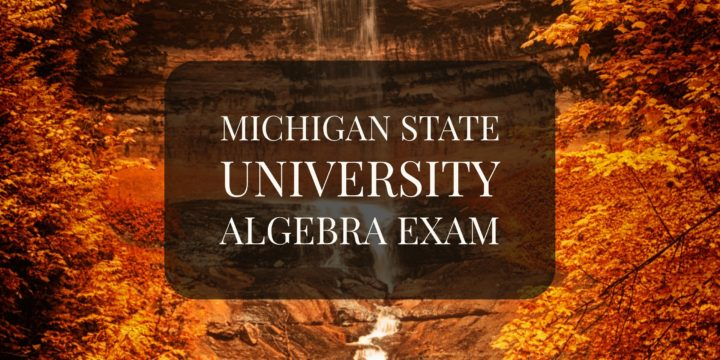# Every Finite Group Having More than Two Elements Has a Nontrivial Automorphism## Problem 495

Prove that every finite group having more than two elements has a nontrivial automorphism.

(Michigan State University, Abstract Algebra Qualifying Exam)Add to solve later

## Proof.

Let $G$ be a finite group and $|G|> 2$.

### Case When $G$ is a Non-Abelian Group

Let us first consider the case when $G$ is a non-abelian group.
Then there exist elements $g, h\in G$ such that $gh\neq hg$.

Consider the map $\phi: G \to G$ defined by sending $x\in G$ to $gxg^{-1}$.
Then it is straightforward to check that $\phi$ is a group homomorphism and its inverse is given by the conjugation by $g^{-1}$.
Hence $\phi$ is an automorphism.

If $\phi=1$, then we have $h=\phi(h)=ghg^{-1}$, and this implies that $gh=hg$.
This contradicts our choice of $g$ and $h$.
Hence $\phi$ is a non-trivial automorphism of $G$.

### Case When $G$ is an Abelian Group

Next consider the case when $G$ is a finite abelian group of order greater than $2$.
Since $G$ is an abelian group the map $\psi:G\to G$ given by $x \mapsto x^{-1}$ is an isomorphism, hence an automorphism.

If $\psi$ is a trivial automorphism, then we have $x=\psi(x)=x^{-1}$.
Thus, $x^2=e$, where $e$ is the identity element of $G$.

#### Sub-Case When $G$ has an Element of Order $\geq 3$.

Therefore, if $G$ has at least one element of order greater than $2$, then $\psi$ is a non-trivial automorphism.

#### Sub-Case When Elements of $G$ has order $\leq 2$.

It remains to consider the case when $G$ is a finite abelian group such that $x^2=e$ for all elements $x\in G$.
In this case, the group $G$ is isomorphic to
$\Zmod{2}\times \Zmod{2}\times \cdots \Zmod{2}=(\Zmod{2})^n.$ Since $|G| > 2$, we have $n>1$.

Then the map $(\Zmod{2})^n\to (\Zmod{2})^n$ defined by exchanging the first two entries
$(x_1, x_2, x_3, \dots, x_n) \mapsto (x_2, x_1, x_3, \dots, x_n)$ is an example of nontrivial automorphism of $G$.

Therefore, in any case, the group $G$ has a nontrivial automorphism.Add to solve later

### More from my site

#### You may also like...

This site uses Akismet to reduce spam. Learn how your comment data is processed.

###### More in Group Theory##### If Two Subsets $A, B$ of a Finite Group $G$ are Large Enough, then $G=AB$

Let $G$ be a finite group and let $A, B$ be subsets of $G$ satisfying $|A|+|B| > |G|.$ Here $|X|$...

Close